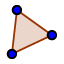# Is Round "In Shape?"

## Definition 19

Rectilinear figures are those which are contained by straight lines, trilateral figures being contained by three lines, quadrilateral figures those contained by four lines, and multilateral figures those contained by more than four straight lines. Recti- comes from the Latin word, rectum (yes, the same word, look at a diagram of intestines and you will see why), which means straight (did you google intestines? The rectum is the straight part at the end of your intestines); so rectilinear figures are made of straight lines. Circles are not rectilinear figures. Trilateral (three lines) figures are what we call triangles today and quadrilateral (four lines) figures are what we call... quadrilaterals, we didn't change that one (technically we should call them quadrangles if we followed the triangle naming, lets see if we can make that catch on!) Multilateral (many lines) figures are what we generally call polygons (many angles in Greek) in high school now.

## Tool Practice

Use thetool to draw different figures, with different side lengths. See if you can create a perfect square... It is possible with the tools we have but it will take being very clever to do it in such a way that even if you drag the points around, the shape stays a square.